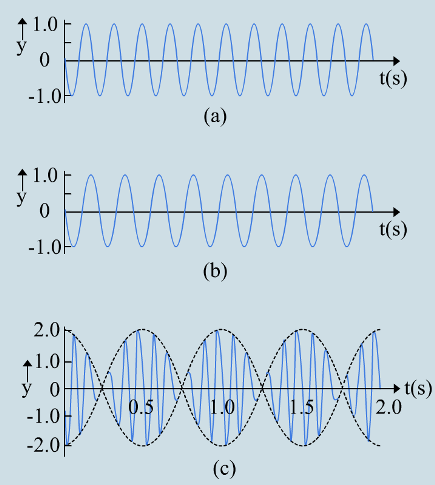Beats Produced by two Tuning Forks

# Our Objective

To demonstrate the phenomenon of beats produced by two tuning forks of slightly different frequencies.

# The Theory

## Definition of beats

When two sounds of slightly different frequencies reach our ears simultaneously, frequency of the combined sound will be the average of frequencies of two sound waves, and we hear periodic variations in the sound. These periodic variations of sound are called beats.

Consider two harmonic sound waves of angular frequencies of W1 and W2

W1 is slightly greater than W2

Displacements of two sound waves respectively are

S= a cos (W1t)

S= a cos (W2t)

When two sounds hear simultaneously resultant displacement by the principle of superposition.

S = S1+S2

= 2 a cos((W1-W2)t/2)   cos((W1+W2)t/2)

W= (W1-W2)/2             Wa = (W1+W2)/2

S = 2 a cos((Wb)t) cos((Wa)t)

W= 2* π * v

W= 2* π * v

W= 2* π * va

W= 2* π * v

Wa – Angular frequency of resultant wave

v1, v2 are frequencies sound waves.

Frequency of resultant wave is va.  Increasing and decreasing of loudness happens at the frequency of vb. It is called beat frequency.

v= v1-v

Example

Sound waves of 11 Hz and 9 Hz give the beats of frequency 2 Hz.Superposition of two harmonic waves, one of frequency 11 Hz (a), and the other of frequency 9 H (b), giving rise to beats of frequency 2 Hz as shown in (c).

# Learning Outcomes

• Students can produce beats using tuning forks.
• Students can understand phenomena of beat.
• Students can understand the relationship between the difference of tuning forks and frequency of beat.